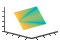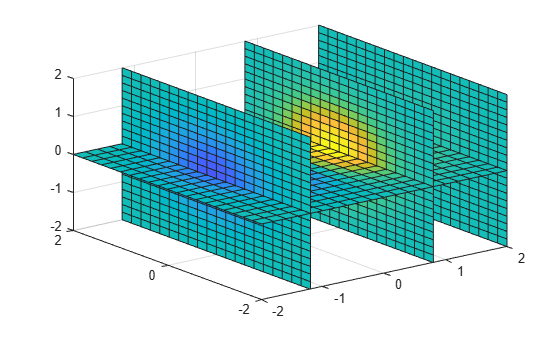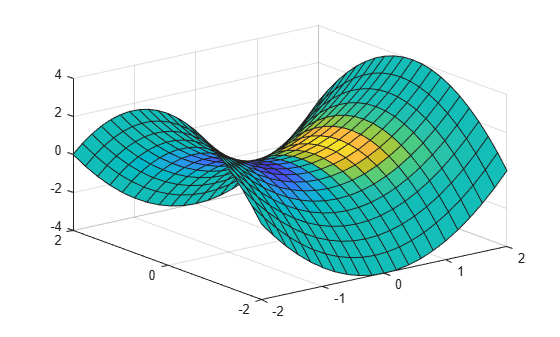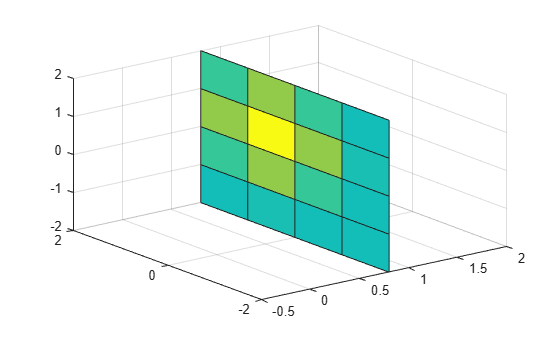# slice

Volume slice planes

•## Syntax

``slice(X,Y,Z,V,xslice,yslice,zslice) ``
``slice(V,xslice,yslice,zslice)``
``slice(___,method) ``
``slice(ax,___)``
``s = slice(___)``

## Description

example

````slice(X,Y,Z,V,xslice,yslice,zslice) `draws slices for the volumetric data `V`. Specify `X`,`Y`, and `Z` as the coordinate data. Specify `xslice`, `yslice`, and `zslice` as the slice locations using one of these forms: To draw one or more slice planes that are orthogonal to a particular axis, specify the slice arguments as a scalar or vector.To draw a single slice along a surface, specify all the slice arguments as matrices that define a surface. ```
````slice(V,xslice,yslice,zslice)` uses the default coordinate data for `V`. The (x,y,z) location for each element in `V` is based on the column, row, and page index, respectively.```

example

````slice(___,method) `specifies the interpolation method, where `method` can be `'linear'` (the default), `'cubic'`, or `'nearest'`. Use this option with any of the input arguments in previous syntaxes.```
````slice(ax,___)` plots into the specified axes, instead of the current axes (`gca`).```
````s = slice(___)` returns the `Surface` objects created. `slice` returns one `Surface` object for each slice.```

## Examples

collapse all

Show volumetric data along slice planes that are orthogonal to each axis.

Create slice planes through the volume defined by $v=x{e}^{-{x}^{2}-{y}^{2}-{z}^{2}}$, where x, y, and z range from `[-2,2]`. Create slice planes orthogonal to the x-axis at the values `-1.2`, `0.8`, and `2` and orthogonal to the z-axis at the value `0`. Do not create any slice planes that are orthogonal to the y-axis by specifying an empty array.

```[X,Y,Z] = meshgrid(-2:.2:2); V = X.*exp(-X.^2-Y.^2-Z.^2); xslice = [-1.2,0.8,2]; yslice = []; zslice = 0; slice(X,Y,Z,V,xslice,yslice,zslice)```Show volumetric data along a nonplanar slice. Define the surface where you want to show the volumetric data.

Create volume array `V` as the volume defined by $v=x{e}^{-{x}^{2}-{y}^{2}-{z}^{2}}$, where x, y, and z range from `[-5,5]`. Then, show a slice of the volume data along the surface defined by $z={x}^{2}-{y}^{2}$.

```[X,Y,Z] = meshgrid(-5:0.2:5); V = X.*exp(-X.^2-Y.^2-Z.^2); [xsurf,ysurf] = meshgrid(-2:0.2:2); zsurf = xsurf.^2-ysurf.^2; slice(X,Y,Z,V,xsurf,ysurf,zsurf)```Create a slice plane through volumetric data. Specify the interpolation method for the data values.

Create a slice plane orthogonal to the x-axis at the value 0.8. Since the volume data is not defined for x values of 0.8, the `slice` function interpolates the nearby values. To use the nearest data point value, specify the interpolation method as `'nearest'`.

```[X,Y,Z] = meshgrid(-2:2); V = X.*exp(-X.^2-Y.^2-Z.^2); xslice = 0.8; yslice = []; zslice = []; slice(X,Y,Z,V,xslice,yslice,zslice,'nearest')```## Input Arguments

collapse all

x-axis coordinate data, specified as a vector or a 3-D array the same size as `V`. If you specify a 3-D array, it must be monotonic and orthogonally spaced, as if produced by the `meshgrid` function.

Data Types: `single` | `double`

y-axis coordinate data, specified as a vector or a 3-D array the same size as `V`. If you specify a 3-D array, it must be monotonic and orthogonally spaced, as if produced by the `meshgrid` function.

Data Types: `single` | `double`

z-axis coordinate data, specified as a vector or a 3-D array the same size as `V`. If you specify a 3-D array, it must be monotonic and orthogonally spaced, as if produced by the `meshgrid` function.

Data Types: `single` | `double`

Volume data, specified as a 3-D array.

Data Types: `single` | `double`

x-axis slice values, specified in one of these forms:

• Scalar — Draw one slice plane orthogonal to the x-axis at the specified location.

• Vector — Draw multiple slice planes orthogonal to the x-axis at the specified locations.

• `[]` — Do not draw any slice planes.

• Matrix — Draw the slice along a surface, instead of a plane. If you use this option, then `yslice` and `zslice` also must be matrices that are the same size.

Example: `[2 4]` draws slice planes orthogonal to the x-axis at the values 2 and 4.

Data Types: `single` | `double`

y-axis slice values, specified in one of these forms:

• Scalar — Draw one slice plane orthogonal to the y-axis at the specified location.

• Vector — Draw multiple slice planes orthogonal to the y-axis at the specified locations.

• `[]` — Do not draw any slice planes.

• Matrix — Draw the slice along a surface, instead of a plane. If you use this option, then `xslice` and `zslice` also must be matrices that are the same size.

Example: `[2 4]` draws slice planes orthogonal to the y-axis at the values 2 and 4.

Data Types: `single` | `double`

z-axis slice values, specified in one of these forms:

• Scalar — Draw one slice plane orthogonal to the z-axis at the specified location.

• Vector — Draw multiple slice planes orthogonal to the z-axis at the specified locations.

• `[]` — Do not draw any slice planes.

• Matrix — Draw the slice along a surface, instead of a plane. If you use this option, then `xslice` and `yslice` also must be matrices that are the same size.

Example: `[2 4]` draws slice planes orthogonal to the z-axis at the values 2 and 4.

Data Types: `single` | `double`

Interpolation method, specified as one of these values:

• `'linear'` — Linear interpolation of the values at neighboring grid points in each respective dimension

• `'cubic'` — Cubic interpolation of the values at neighboring grid points in each respective dimension

• `'nearest'` — Nearest grid point value

Target axes, specified as an `Axes` object. If you do not specify the axes, then `slice` plots into the current axes.

## Version History

Introduced before R2006a